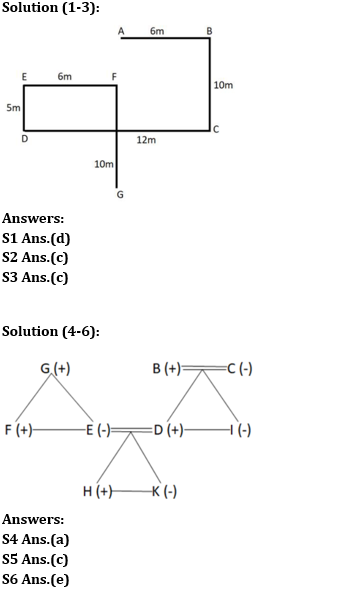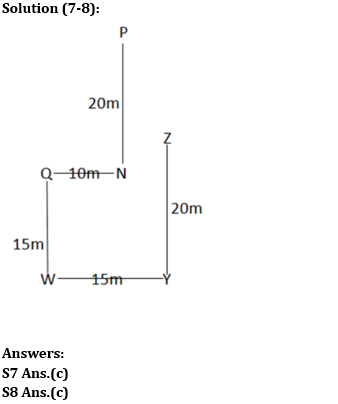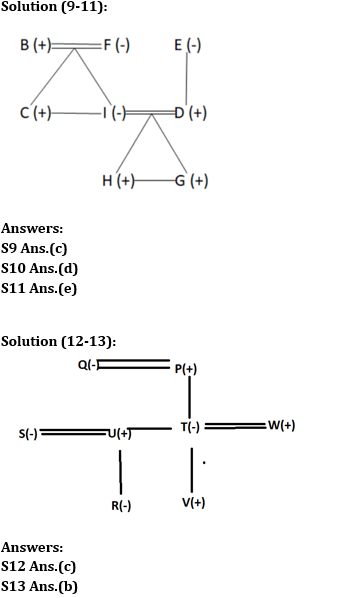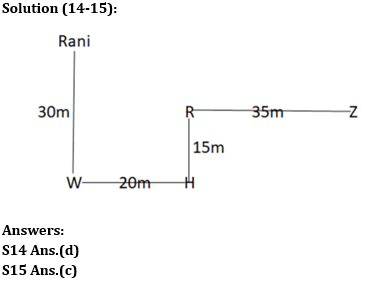Latest Banking jobs   »

# Reasoning Ability Quiz For RBI Grade B/ ECGC PO/ SIDBI Grade A Prelims 2022- 15th April

Directions (1-4): Study the information carefully and answer the questions accordingly.
Point A is 6m west of point B. Point B is 10m north of point C. Point D is 5m south of point E and 12m west of point C. Point E is 6m west of point F. Point F is 10m north of point G.

Q1. Point F is in which direction of point B?
(a) South
(b) North
(c) South – east
(d) South – west
(e) None of these

Q2. What is the shortest distance between the points A and F?
(a) 7m
(b) 6m
(c) 5m
(d) 4m
(e) None of these

Q3. Point E is in which direction of point C?
(a) North
(b) South
(c) North-west
(d) North-east
(e) None of these

Direction (4-6): Study the information carefully and answer the questions accordingly.
There are nine members in a family with two married couples and three generations. B is the grandfather of H who is the nephew of I. K is the daughter of E. D is the brother-in-law of F who is unmarried. G is the father of E. There are four females. C is the mother of I who is the sister-in-law of E. I is unmarried. B has only one daughter.

Q4. How is C related to K?
(a) Grandmother
(b) Sister
(c) Aunt
(d) Mother
(e) None of these

Q5. How is I related to H?
(a) Uncle
(b) Mother
(c) Aunt
(d) Sister
(e) None of these

Q6. Find the correct statement from the following?
(a) G is the father of I
(b) H and K are not siblings
(c) E is the sister-in-law of F
(d) B is the father of F
(e) E is mother of H

Directions (7-8): Study the following information carefully and answer the questions accordingly.
Point P is 20m north of point N. Point Y is 20m south of point Z and 15m east of point W. Point Q is 10m west of point N. Point W is 15m south of point Q.

Q7. Point Q is in which direction of point Z?
(a) South
(b) North-East
(c) South-West
(d) West
(e) None of these

Q8. What is the shortest distance between points Z and N?
(a) 12m
(b) 5√7m
(c) 5√2m
(d) 9m
(e) None of these

Direction (9-11): Study the information carefully and answer the questions accordingly.
B is the father of C who is the brother-in-law of D. F has a son and a daughter. G and H are the grandsons of E. F is the mother-in-law of D. There are at most five males. I is the daughter-in-law of E who has only one child. D is the son of E.

Q9. How many females are there?
(a) Two
(b) Five
(c) Three
(d) Four
(e) None of these

Q10. How is B related to H?
(a) Uncle
(b) Nephew
(c) Son
(d) Grandfather
(e) None of these

Q11. Four from the following five are similar in a certain way and forms a group. Find out the one who does not belong to that group?
(a) H
(b) G
(c) B
(d) C
(e) F

Directions (12-13): Read the following information carefully and answer the questions given below.
There are eight members i.e., P, Q, R, S, T, U, V, and W in a family of three generations and there are three married couples. R is an unmarried person. Q is the mother-in-law of S. U is the brother-in-law of W who has no sibling. T is the aunt of R who is the daughter of U. U is the child of P who has one granddaughter and one grandson. U has only one sister and no brother. W is the father of V.

Q12. What is the relation of V with respect to P?
(a) Son
(b) Son-in-law
(c) Grandson
(d) Brother-in-law
(e) None of these

Q13. Who amongst the following person is the sister-in-law of T?
(a) Q
(b) S
(c) R
(d) Cannot be determined
(e) None of these

Directions (14-15): Study the information carefully and answer the questions accordingly.

Rani starts walking towards the south to reach point W after a distance of 30m. Now, she took a left turn and reached point H after a distance of 20m. From here, she took a left turn and covered a distance of 15m to reach point R. Finally, she turned towards the east and covered a distance of 35m to reach point Z.

Q14. What is the shortest distance between the points W and Z?
(a) 65m
(b) 5√110m
(c) 40m
(d) 5√130m
(e) None of these

Q15.Point H is in which direction of Rani’s starting point?
(a) South
(b) West
(c) South-East
(d) North-West
(e) None of these

Solutions